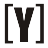# Amps (A) to Volts (V) Calculator

To calculate Amps to Volts, select with (watts or Ω) first, insert value of amps and (watts or (Ω) ohms) then press Calculate Button to get the result of volts (V). Swap to Volts to Amps calculator.

 Select (watts/ohms): (A) to (V) with watts (A) to (V) with ohms Enter amps: A Enter watts: W Result in volts: V

## How to Calculate Amps (A) to Volts (V)

(A) to (V) Formula
`V (V) = P (W) / I (A)`

Voltage in volts (V) equal to the power P in watts (W) and divided by the current I in amps (A).

`V (V) = I (A) × R (Ω)`

Voltage in volts (V) equal to the current I in amps (A), multiply by the resistance R in ohms (Ω).YoosFuhl.com Would you like to receive notifications on latest updates? No Yes